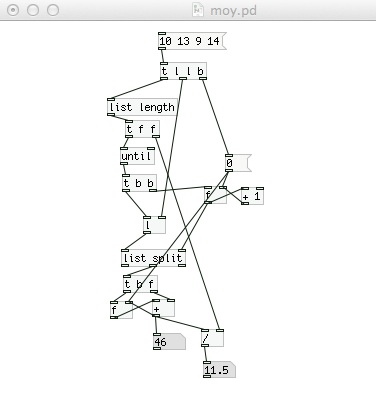• ### Finding the average of a set of numbers!

Hi guys, I'm running Pd 0.46.5 so I can't use the average object. My question is:

I have a stream of numbers coming in which I want to find the average of. I want to do this by adding each number to the next, and then dividing it by the total of numbers being added together. I'm struggling to implement this though, can someone help?

So far I've made a counter to count how many numbers there are to divide by, but I don't know how to write the expression to calculate the average.

Regards

• Posts 3 | Views 1579